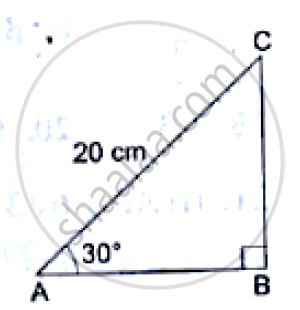# In the Adjoining Figure, δAbc is a Right-angled Triangle in Which ∠B = 900, ∠300 and Ac = 20cm. Find (I) Bc, (Ii) Ab. - Mathematics

In the adjoining figure, ΔABC is a right-angled triangle in which ∠B = 900, ∠300 and AC = 20cm. Find (i) BC, (ii) AB.

#### Solution

From the given right-angled triangle, we have:(BC)/(AC) = sin 30^0

⇒(BC)/20=1/2

⇒BC = 20/2 = 10cm

Also, (AB)/(AC) = cos 30^0

⇒(AB)/20=sqrt(3)/2

⇒AB = (20xxsqrt(3)/2) = 10sqrt(3) cm

∴ BC = 10cm and AB = 10sqrt(3) cm

Concept: Trigonometric Ratios and Its Reciprocal
Is there an error in this question or solution?

#### APPEARS IN

RS Aggarwal Secondary School Class 10 Maths
Chapter 6 T-Ratios of some particular angles
Exercises | Q 20
Share